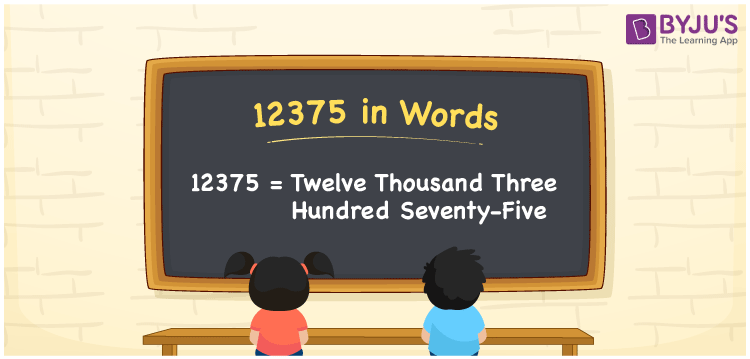# 12375 in Words

We can write the number 12375 in words as Twelve thousand three hundred seventy-five. If you spent Rs. 12375 to buy a microwave oven, you could say, “I spent Twelve thousand three hundred seventy-five rupees on buying a microwave oven”. Also, we know 12375 is a cardinal number since it represents a certain quantity.

 12375 in words Twelve thousand three hundred seventy-five Twelve thousand three hundred seventy-five in Numbers 12375

## 12375 in English Words

Generally, we use English alphabets to write numbers in words, and thus, we spell 12375 in English words as “Twelve thousand three hundred seventy-five”.## How to Write 12375 in Words?

The number 12375 contains five digits. Let us make a place value chart with five columns since the number 12375 is a five-digit number.

 Ten thousands Thousands Hundreds Tens Ones 1 2 3 7 5

Here, ones = 5, tens = 7, hundreds = 3, thousands = 2, ten thousands = 1

The above numbers can be expanded as follows.

So, 1 × Ten thousand + 2 × Thousand + 4 × Hundred + 0 × Ten + 0 × One

= 1 × 10000 + 2 × 1000 + 3 × 100 + 7 × 10 + 5 × 1

= 10000 + 2000 + 300 + 70 + 5

= Ten Thousand + Two thousand + Three hundred + Seventy + Five

= Twelve thousand three hundred seventy-five

Therefore, 12375 in words = Twelve thousand three hundred seventy-five.

12375 is a natural number that is the successor of 12374 and predecessor of 12376.

12375 in words – Twelve thousand three hundred seventy-five

Is 12375 an even number? – No

Is 12375 an odd number? – Yes

Is 12375 a prime number? – No

Is 12375 a composite number? – Yes

Is 12375 a perfect square number? – No

Is 12375 a perfect cube number? – No

## Frequently Asked Questions on 12375 in Words

Q1

### How do you write 12375 in words?

The number 12375 can be written in words as Twelve thousand three hundred seventy-five.
Q2

### How to write Rs. 12375 on a cheque?

On a cheque, we generally write Rs. 12375 in words as “Twelve thousand three hundred seventy-five rupees only”.
Q3

### Write the expanded form of 12375 in words.

12375 = 12000 + 300 + 70 + 5 = 1 × 10000 + 2 × 1000 + 3 × 100 + 7 × 10 + 5 × 1 = 1 × Ten thousand + 2 × Thousand + 3 × Hundred + 7 × Ten + 5 × One